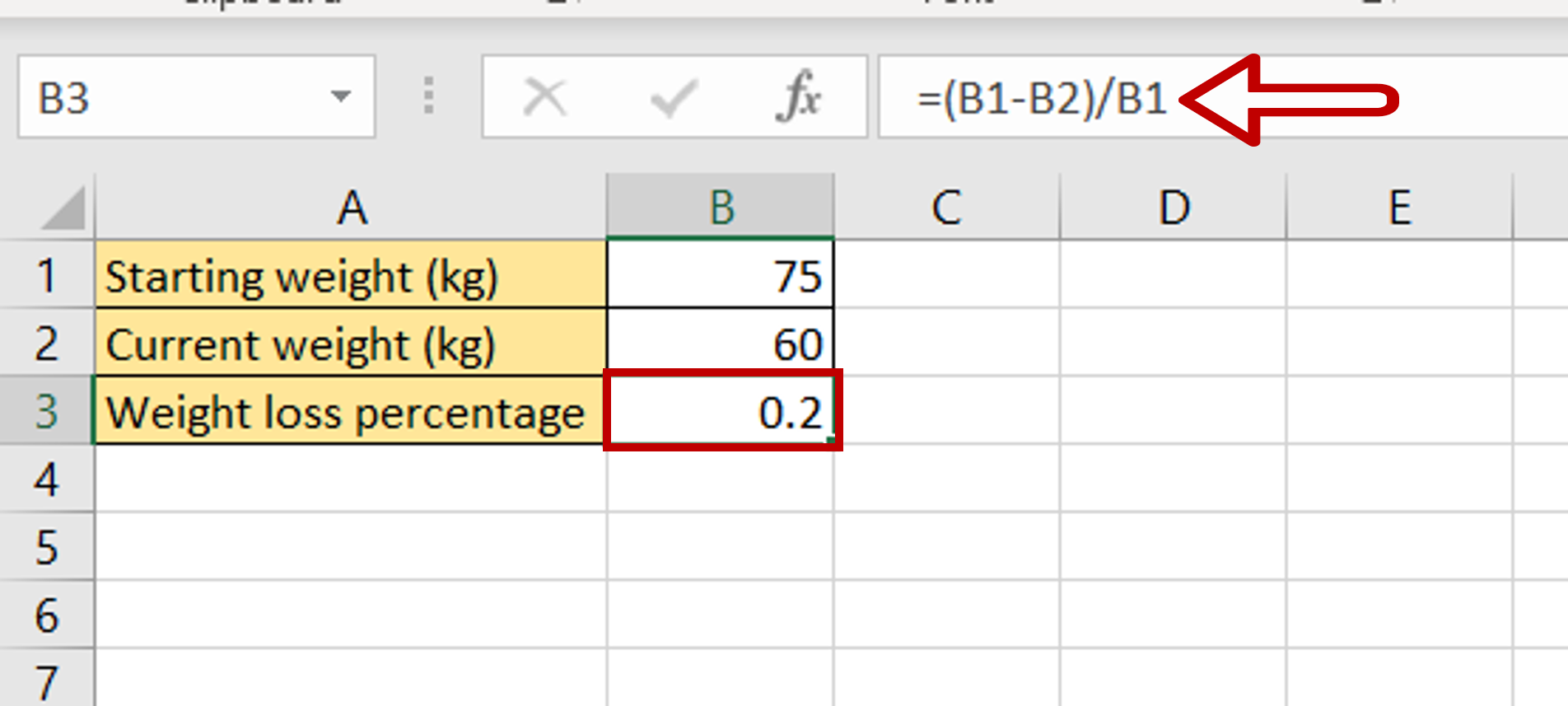# How to calculate the percentage of weight loss in Excel

You can watch a video tutorial here.Excel can be used for all types of calculations and can be used to create formulas. When creating a formula, it is better to use cell references so that the formula is dynamic and will be updated whenever the source values change. The percentage of weight loss can be computed using the starting weight and the current weight.

The formula is as follows:

Percentage of weight loss = ((Starting weight-Current weight)/Starting weight)*100

### Step 1 – Create the formula– Select the cell where the result is to appear
– Type the formula using cell references:
= (Starting weight (kg) – Current weight (kg)) / Starting weight (kg)

### Step 2 – Check the result– Select the computed value and click the Percent Style button on the ribbon
– The weight loss percentage is displayed